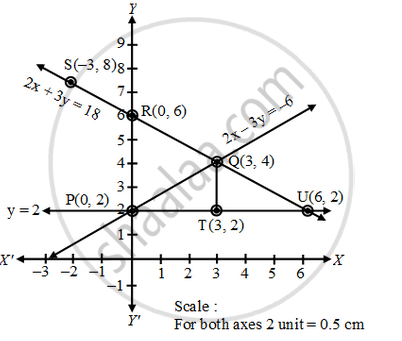# Draw the graphs of the following equations 2x – 3y = – 6; 2x + 3y = 18; y = 2 Find the vertices of the triangles formed and also find the area of the triangle. - Mathematics

Sum

Draw the graphs of the following equations 2x – 3y = – 6; 2x + 3y = 18; y = 2 Find the vertices of the triangles formed and also find the area of the triangle.

#### Solution

(a) Graph of the equation 2x – 3y = – 6;

We have, 2x – 3y = – 6 ⇒ y = \frac { 2x+6 }{ 3 }

When, x = 0, y = 2

When, x = 3, y = 4

Plotting the points P(0, 2) and Q(3, 4) on the graph paper and drawing a line joining between them we get the graph of the equation 2x – 3y = – 6 as shown in fig.

(b) Graph of the equation 2x + 3y = 18;

We have 2x + 3y = 18 ⇒ y = \frac { -2x+18 }{ 3 }

When, x = 0, y = 6

When, x = – 3, y = 8

Plotting the points R(0, 6) and S(–3, 8) on the same graph paper and drawing a line joining between them, we get the graph of the equation 2x + 3y = 18 as shown in fig

(c) Graph of the equation y = 2

It is a clear fact that y = 2 is for every value of x. We may take the points T (3, 2), U(6, 2) or any other values. Plotting the points T(3, 2) and U(6, 2) on the same graph paper and
drawing a line joining between them, we get the graph of the equation y = 2 as shown in figFrom the fig., we can observe that the lines taken in pairs intersect each other at points Q(3, 4), U (6, 2) and P(0, 2). These form the three vertices of the triangle PQU.

To find area of the triangle so formed

The triangle is so formed is PQU (see fig.)

In the ∆PQU

QT (altitude) = 2 units and PU (base) = 6 units

so, area of ∆PQU = (base × height)

= 1/2 (PU × QT) = 1/2 × 6 × 2 sq. untis

= 6 sq. units.

Concept: Graphical Method of Solution of a Pair of Linear Equations
Is there an error in this question or solution?